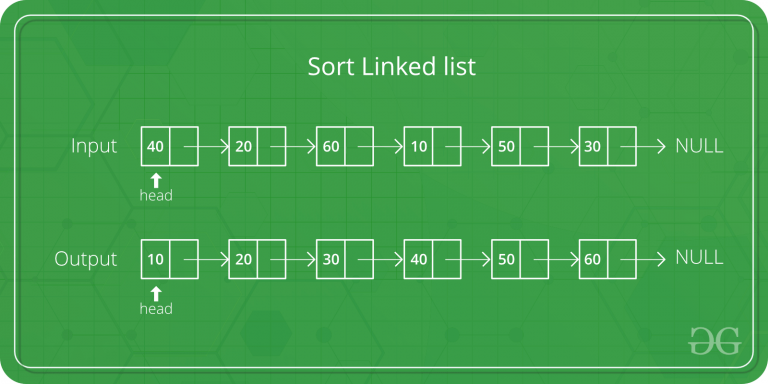Related Articles

# C Program for Merge Sort for Linked Lists

• Difficulty Level : Hard
• Last Updated : 21 Oct, 2021

Merge sort is often preferred for sorting a linked list. The slow random-access performance of a linked list makes some other algorithms (such as quicksort) perform poorly, and others (such as heapsort) completely impossible.Let head be the first node of the linked list to be sorted and headRef be the pointer to head. Note that we need a reference to head in MergeSort() as the below implementation changes next links to sort the linked lists (not data at the nodes), so head node has to be changed if the data at original head is not the smallest value in linked list.

```MergeSort(headRef)
1) If head is NULL or there is only one element in the Linked List
then return.
2) Else divide the linked list into two halves.
FrontBackSplit(head, &a, &b); /* a and b are two halves */
3) Sort the two halves a and b.
MergeSort(a);
MergeSort(b);
4) Merge the sorted a and b (using SortedMerge() discussed here)
```

## C

 `// C code for linked list merged sort``#include ``#include `` ` `/* Link list node */``struct` `Node {``    ``int` `data;``    ``struct` `Node* next;``};`` ` `/* function prototypes */``struct` `Node* SortedMerge(``struct` `Node* a, ``struct` `Node* b);``void` `FrontBackSplit(``struct` `Node* source,``                    ``struct` `Node** frontRef, ``struct` `Node** backRef);`` ` `/* sorts the linked list by changing next pointers (not data) */``void` `MergeSort(``struct` `Node** headRef)``{``    ``struct` `Node* head = *headRef;``    ``struct` `Node* a;``    ``struct` `Node* b;`` ` `    ``/* Base case -- length 0 or 1 */``    ``if` `((head == NULL) || (head->next == NULL)) {``        ``return``;``    ``}`` ` `    ``/* Split head into 'a' and 'b' sublists */``    ``FrontBackSplit(head, &a, &b);`` ` `    ``/* Recursively sort the sublists */``    ``MergeSort(&a);``    ``MergeSort(&b);`` ` `    ``/* answer = merge the two sorted lists together */``    ``*headRef = SortedMerge(a, b);``}`` ` `/* See https:// www.geeksforgeeks.org/?p=3622 for details of this ``function */``struct` `Node* SortedMerge(``struct` `Node* a, ``struct` `Node* b)``{``    ``struct` `Node* result = NULL;`` ` `    ``/* Base cases */``    ``if` `(a == NULL)``        ``return` `(b);``    ``else` `if` `(b == NULL)``        ``return` `(a);`` ` `    ``/* Pick either a or b, and recur */``    ``if` `(a->data <= b->data) {``        ``result = a;``        ``result->next = SortedMerge(a->next, b);``    ``}``    ``else` `{``        ``result = b;``        ``result->next = SortedMerge(a, b->next);``    ``}``    ``return` `(result);``}`` ` `/* UTILITY FUNCTIONS */``/* Split the nodes of the given list into front and back halves,``    ``and return the two lists using the reference parameters.``    ``If the length is odd, the extra node should go in the front list.``    ``Uses the fast/slow pointer strategy. */``void` `FrontBackSplit(``struct` `Node* source,``                    ``struct` `Node** frontRef, ``struct` `Node** backRef)``{``    ``struct` `Node* fast;``    ``struct` `Node* slow;``    ``slow = source;``    ``fast = source->next;`` ` `    ``/* Advance 'fast' two nodes, and advance 'slow' one node */``    ``while` `(fast != NULL) {``        ``fast = fast->next;``        ``if` `(fast != NULL) {``            ``slow = slow->next;``            ``fast = fast->next;``        ``}``    ``}`` ` `    ``/* 'slow' is before the midpoint in the list, so split it in two``    ``at that point. */``    ``*frontRef = source;``    ``*backRef = slow->next;``    ``slow->next = NULL;``}`` ` `/* Function to print nodes in a given linked list */``void` `printList(``struct` `Node* node)``{``    ``while` `(node != NULL) {``        ``printf``(``"%d "``, node->data);``        ``node = node->next;``    ``}``}`` ` `/* Function to insert a node at the beginging of the linked list */``void` `push(``struct` `Node** head_ref, ``int` `new_data)``{``    ``/* allocate node */``    ``struct` `Node* new_node = (``struct` `Node*)``malloc``(``sizeof``(``struct` `Node));`` ` `    ``/* put in the data */``    ``new_node->data = new_data;`` ` `    ``/* link the old list off the new node */``    ``new_node->next = (*head_ref);`` ` `    ``/* move the head to point to the new node */``    ``(*head_ref) = new_node;``}`` ` `/* Driver program to test above functions*/``int` `main()``{``    ``/* Start with the empty list */``    ``struct` `Node* res = NULL;``    ``struct` `Node* a = NULL;`` ` `    ``/* Let us create a unsorted linked lists to test the functions``Created lists shall be a: 2->3->20->5->10->15 */``    ``push(&a, 15);``    ``push(&a, 10);``    ``push(&a, 5);``    ``push(&a, 20);``    ``push(&a, 3);``    ``push(&a, 2);`` ` `    ``/* Sort the above created Linked List */``    ``MergeSort(&a);`` ` `    ``printf``(``"Sorted Linked List is: \n"``);``    ``printList(a);`` ` `    ``getchar``();``    ``return` `0;``}`
Output:
```Sorted Linked List is:
2 3 5 10 15 20
```

Time Complexity: O(n*log n)

Space Complexity: O(n*log n)

Please refer complete article on Merge Sort for Linked Lists for more details!

We provide nothing but the best curated videos and practice problems for our students. Check out the C Foundation Course and master the C language from basic to advanced level. Wait no more, start learning today!

My Personal Notes arrow_drop_up